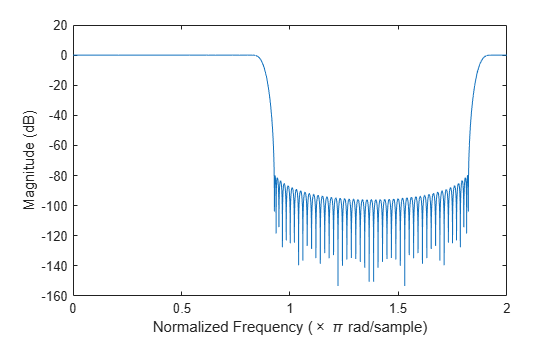# freqz

Frequency response of filters in channelizer

## Syntax

[H,w] = freqz(obj)
[H,w] = freqz(obj,ind)
[H,f] = freqz(obj,ind,Name,Value)

## Description

example

[H,w] = freqz(obj) computes a matrix of complex frequency responses for each filter in the dsp.Channelizer System object™. Each column of H corresponds to the frequency response for one of the filters in the channelizer. w is a vector of normalized frequencies at which the rows of H are computed.

example

[H,w] = freqz(obj,ind) computes the frequency response of the filters with indices corresponding to the elements in the vector ind. ind is a row vector of indices between 1 and obj.NumFrequencyBands. By default, this vector is [1:N], where N is the number of frequency bands.For example, to compute the frequency response of the first 4 filters, set ind to [1:4].channelizer = dsp.Channelizer; [H,w] = freqz(channelizer,[1:4]);

example

[H,f] = freqz(obj,ind,Name,Value) computes the frequency response of the filters with additional options specified by one or more Name,Value pair arguments.For example, to specify a sampling rate of 44100 Hz, set 'Fs' to 44100. To compute the frequency response using 1024 frequency points, set 'NFFT' to 1024. In addition, to compute the sum of the frequency response of the filters, set 'overall' to true.channelizer = dsp.Channelizer; [H,f] = freqz(channelizer,[1:4],'Fs',44100,'NFFT',1024,'overall',true);

## Examples

collapse all

Compute the frequency response of the filters in the channelizer using the freqz function.

Design a channelizer with the number of frequency bands or polyphase branches set to 8, the number of taps or coefficients per band set to 12, and stopband attenuation set to 80 dB. Compute the frequency response matrix, H, and the corresponding vector of frequency points, w.

channelizer = dsp.Channelizer; [H,w] = freqz(channelizer); %#ok whos H
 Name Size Bytes Class Attributes H 8192x8 1048576 double complex 

The number of rows in H corresponds to the number of frequency points, and the number of columns in H corresponds to the number of frequency bands. To view only a portion of the filter bank, specify the indices.

[H,w] = freqz(channelizer,(1:4)); %#ok

Specifying the filter indices as [1:4] computes the individual frequency response of the first 4 filters. You can alternatively view the sum of the filter responses by setting the 'overall' to true.

[H,w] = freqz(channelizer,1:4,'overall',true); plot(w/pi,20*log10(abs(H))) xlabel('Normalized Frequency (\times \pi rad/sample)') ylabel('Magnitude (dB)')You can also compute the frequencies in Hz by passing a sampling frequency. Frequency in Hz, f, equals $\left(w/2*pi\right)*Fs$, where w is frequency in radians, and Fs is the sampling rate.

[H,f] = freqz(channelizer,'Fs',44100); %#ok

Specify the number of frequency points using the 'NFFT' argument.

[H,f] = freqz(channelizer,'Fs',44100,'NFFT',1024);

## Input Arguments

collapse all

Input filter, specified as a dsp.Channelizer System object.

Example: [H,w] = freqz(channelizer);

Filter indices, specified as a row vector in the range [1 obj.NumFrequencyBands]. By default, ind is set to 1:N, where N is the number of frequency bands specified through the obj.NumFrequencyBands property.

Example: freqz(channelizer,[1:4]);

Data Types: single | double | int8 | int16 | int32 | int64 | uint8 | uint16 | uint32 | uint64

### Name-Value Arguments

Specify optional pairs of arguments as Name1=Value1,...,NameN=ValueN, where Name is the argument name and Value is the corresponding value. Name-value arguments must appear after other arguments, but the order of the pairs does not matter.

Before R2021a, use commas to separate each name and value, and enclose Name in quotes.

Example: [H,f] = freqz(channelizer,[1:4],'Fs',44100,'NFFT',1024,'overall',true);

Sampling rate, specified as a scalar. This value determines the frequencies in Hz at which freqz computes the frequency response.

Example: 44100

Example: 22050

Data Types: single | double | int8 | int16 | int32 | int64 | uint8 | uint16 | uint32 | uint64

Number of frequency points used to compute the frequency response, specified as a positive scalar.

Example: 8192

Example: 1024

Data Types: single | double | int8 | int16 | int32 | int64 | uint8 | uint16 | uint32 | uint64

The type of filter response, specified as either:

• true –– freqz computes the sum of the filter responses.

• false –– freqz computes the individual filter responses.

Data Types: logical

## Output Arguments

collapse all

Complex frequency response of the filters in the channelizer. The dimensions of the output depend on the value of the 'overall' argument:

• When the 'overall' argument is true, the frequency response vector contains the sum of the frequency responses of all the filters. The vector is of size [nfft 1], where nfft is the number of frequency points. For example, if nfft is 8192, H is a matrix of size [8192 1].

• When the 'overall' argument is false, the frequency response is a matrix of size [nfft nFilters], where nfft is the number of frequency points and nFilters is the number of filters whose frequency response is computed. Suppose nfft is 8192 and ind is [2:5], H is a matrix of size [8192 4].

Data Types: double
Complex Number Support: Yes

Normalized frequencies, specified in rad/sample, at which the frequency response is computed. The vector is of size [nfft 1].

Data Types: double

Frequencies, specified in Hz, at which the frequency response is computed. The vector is of size [nfft 1].

Data Types: double

## Version History

Introduced in R2017b